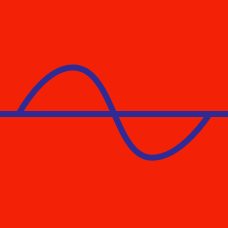Geometry

Graphical Transformation of Trigonometric Functions

Given the graph $y = \tan x$, how do we obtain the graph of $y = 8 \tan x$?

Which of the following is equal to $\cos x?$

Find the maximum value $a$ and the minimum value $b$ of the function $y = \cos x+7.$

Given the graph $y = \cos x$, how do we obtain the graph of $y = \cos 9 x$?

How are the graphs $y_ 1 = \sin ( 2 x)$ and $y_2 = 5 \sin x$ related?

×